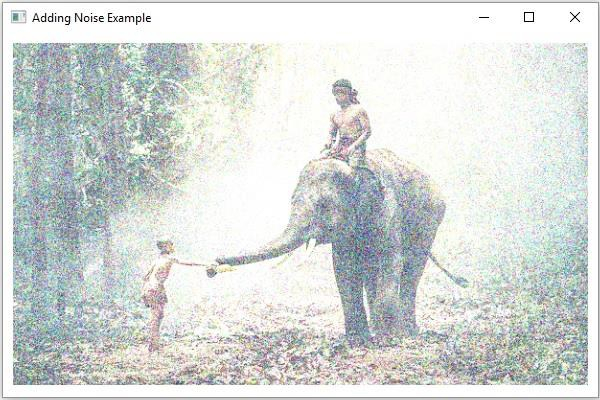# How to add noise to an image using Java OpenCV library?

To add noise to a given image using OpenCV −

• Read the contents of the given image to a Mat object.

• Create two more empty matrices to store the noise and the resultant matrices.

• Create two MatOfDouble matrices to store mean and standard deviation.

• Get the mean and standard deviation values using the meanStdDev() method.

• Create a matrix with random elements (to store the noise) using the randn() method.

• To this method pass the above-created source, mean and standard deviation objects.

Finally, add the noise matrix and source matrix and save as destination.

## Example

import java.awt.Image;
import java.awt.image.BufferedImage;
import java.io.IOException;
import javafx.application.Application;
import javafx.embed.swing.SwingFXUtils;
import javafx.scene.Group;
import javafx.scene.Scene;
import javafx.scene.image.ImageView;
import javafx.scene.image.WritableImage;
import javafx.stage.Stage;
import org.opencv.core.Core;
import org.opencv.core.Mat;
import org.opencv.core.MatOfDouble;
import org.opencv.highgui.HighGui;
import org.opencv.imgcodecs.Imgcodecs;
public class AddingNoise extends Application {
public void start(Stage stage) throws IOException {
//Reading the Image from the file
String file ="D://images//elephant.jpg";
//Creating destination matrix
Mat dst = new Mat(src.rows(), src.cols(), src.type());
//Creating a matrix for the noise
Mat noise = new Mat(src.rows(), src.cols(), src.type());
//Calculating the mean and standard deviation
MatOfDouble mean = new MatOfDouble();
MatOfDouble dev = new MatOfDouble();
Core.meanStdDev(src, mean, dev);
//Filling the noise matrix
Core.randn(noise, mean.get(0,0), dev.get(0,0));
//Converting matrix to JavaFX writable image
Image img = HighGui.toBufferedImage(dst);
WritableImage writableImage= SwingFXUtils.toFXImage((BufferedImage) img, null);
//Setting the image view
ImageView imageView = new ImageView(writableImage);
imageView.setX(10);
imageView.setY(10);
imageView.setFitWidth(575);
imageView.setPreserveRatio(true);
//Setting the Scene object
Group root = new Group(imageView);
Scene scene = new Scene(root, 595, 400);
stage.setScene(scene);
stage.show();
}
public static void main(String args[]) {
launch(args);
}
}

## Input Image## Output

On executing, the above program generates the following output −